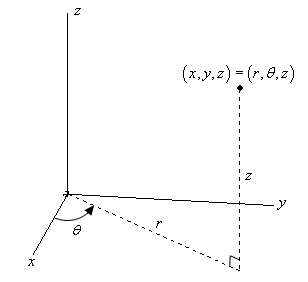Paul's Online Notes
Home / Calculus II / 3-Dimensional Space / Cylindrical Coordinates
Show Mobile Notice Show All Notes Hide All Notes
Mobile Notice
You appear to be on a device with a "narrow" screen width (i.e. you are probably on a mobile phone). Due to the nature of the mathematics on this site it is best views in landscape mode. If your device is not in landscape mode many of the equations will run off the side of your device (should be able to scroll to see them) and some of the menu items will be cut off due to the narrow screen width.

### Section 12.12 : Cylindrical Coordinates

As with two dimensional space the standard $$\left( {x,y,z} \right)$$ coordinate system is called the Cartesian coordinate system. In the last two sections of this chapter we’ll be looking at some alternate coordinate systems for three dimensional space.

We’ll start off with the cylindrical coordinate system. This one is fairly simple as it is nothing more than an extension of polar coordinates into three dimensions. Not only is it an extension of polar coordinates, but we extend it into the third dimension just as we extend Cartesian coordinates into the third dimension. All that we do is add a $$z$$ on as the third coordinate. The $$r$$ and $$\theta$$ are the same as with polar coordinates.

Here is a sketch of a point in $${\mathbb{R}^3}$$.The conversions for $$x$$ and $$y$$ are the same conversions that we used back when we were looking at polar coordinates. So, if we have a point in cylindrical coordinates the Cartesian coordinates can be found by using the following conversions.

\begin{align*}x & = r\cos \theta \\ y & = r\sin \theta \\ z & = z\end{align*}

The third equation is just an acknowledgement that the $$z$$-coordinate of a point in Cartesian and polar coordinates is the same.

Likewise, if we have a point in Cartesian coordinates the cylindrical coordinates can be found by using the following conversions.

\begin{align*}r & = \sqrt {{x^2} + {y^2}} \hspace{0.5in}{\mbox{OR}}\hspace{0.5in}{r^2} = {x^2} + {y^2}\\ \theta & = {\tan ^{ - 1}}\left( {\frac{y}{x}} \right)\\ z & = z\end{align*}

Let’s take a quick look at some surfaces in cylindrical coordinates.

Example 1 Identify the surface for each of the following equations.
1. $$r = 5$$
2. $${r^2} + {z^2} = 100$$
3. $$z = r$$
Show All Solutions Hide All Solutions
a $$r = 5$$ Show Solution

In two dimensions we know that this is a circle of radius 5. Since we are now in three dimensions and there is no $$z$$ in equation this means it is allowed to vary freely. So, for any given $$z$$ we will have a circle of radius 5 centered on the $$z$$-axis.

In other words, we will have a cylinder of radius 5 centered on the $$z$$-axis.

b $${r^2} + {z^2} = 100$$ Show Solution

This equation will be easy to identify once we convert back to Cartesian coordinates.

\begin{align*}{r^2} + {z^2} & = 100\\ {x^2} + {y^2} + {z^2} & = 100\end{align*}

So, this is a sphere centered at the origin with radius 10.

c $$z = r$$ Show Solution

Again, this one won’t be too bad if we convert back to Cartesian. For reasons that will be apparent eventually, we’ll first square both sides, then convert.

\begin{align*}{z^2} & = {r^2}\\ {z^2} & = {x^2} + {y^2}\end{align*}

From the section on quadric surfaces we know that this is the equation of a cone.